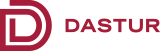### Analytics

Market analysis, optimizations, scenario analysis and decision-making can be enhanced through mathematical and statistical methods. DBTC applies rigorous analytics to understand patterns and to gather insights into the operations of a system. DBTC experts use analytics for analyzing demand-supply dynamics, pricing, market side optimizations and portfolio analysis, to name a few. We use predictive analytics to forecast future behavior and outcomes.

DBTC uses a variety of mathematical techniques as a part of its analytics practice. Some of the indicative areas are:

•Regression models
•Time series
•Experimental designs
•Multivariable statistical process control
•Clustering and segmentation models
•Machine learning

DBTC uses industry standard platforms like R, SAS, Mathematica and MATLAB as the model execution engines.